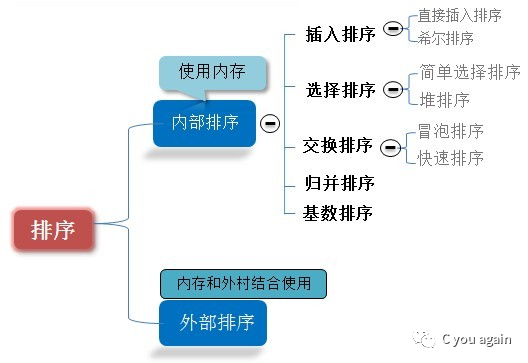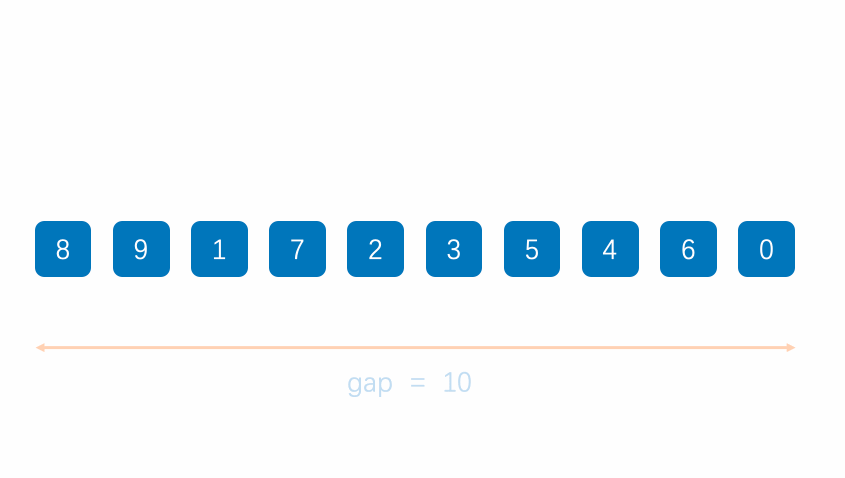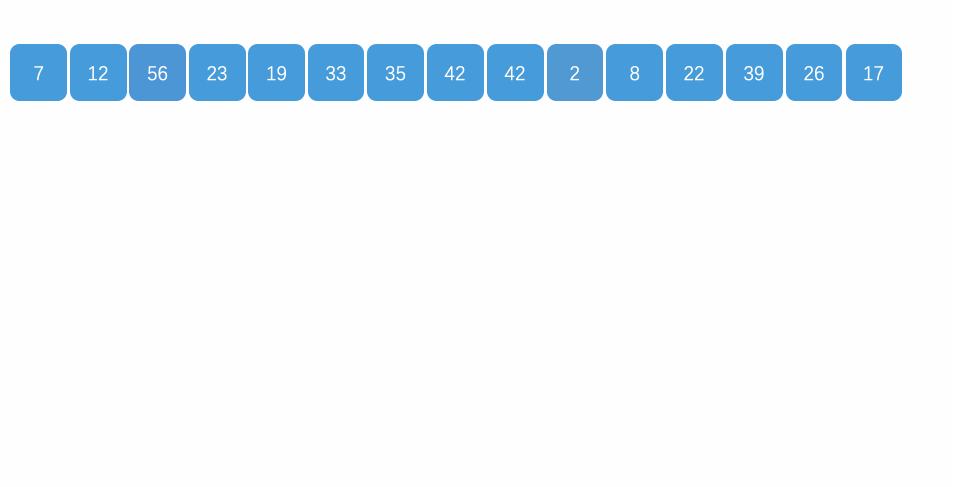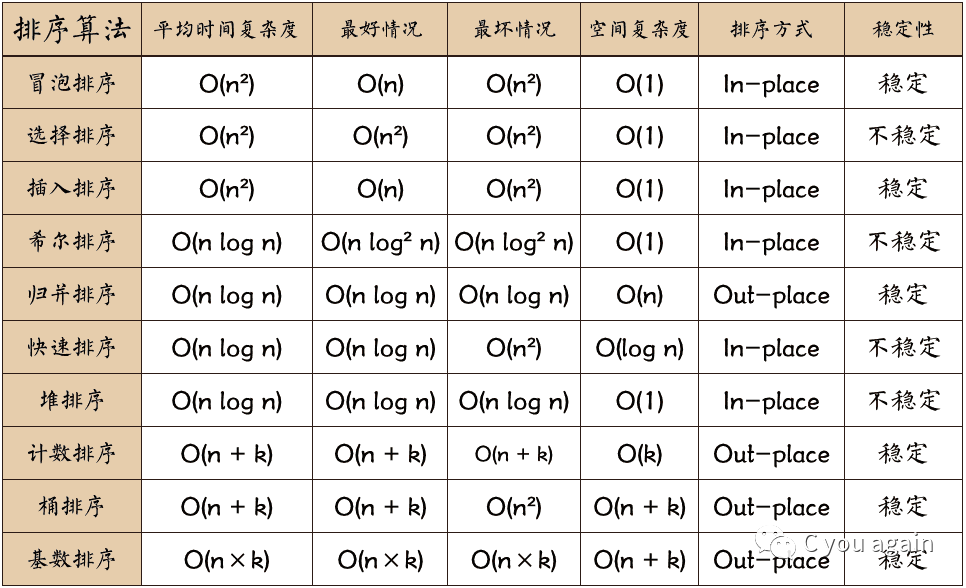# 面试官：手撕十大排序算法，你会几种？

## 演示地址：点击查看演示

《深入浅出理解动态规划（一） | 交叠子问题》

《深入浅出理解动态规划（二） | 最优子结构》

《用x种方式求第n项斐波那契数，99%的人只会第一种》

今天我们就来讨论面试官最喜欢问到的排序算法吧，从冒泡排序、选择排序、插入排序等十大排序算法的排序步骤、代码实现两个方面入手，彻底搞清实现原理，保证面试道路一路畅通。

## 01 排序算法的概述

所谓排序算法，就是通过特定的算法因式将一组或多组数据按照一定模式进行重新排序。

这种新序列遵循着一定的规则，体现出一定的规律，因此，经处理后的数据便于筛选和计算，大大提高了计算效率。

## 02 排序算法的分类## 03评价标准

（1）时间复杂度：即从序列的初始状态到经过排序算法的变换移位等操作变到最终排序好的结果状态的过程所花费的时间度量。

（2）空间复杂度：就是从序列的初始状态经过排序移位变换的过程一直到最终的状态所花费的空间开销。

（3）稳定性：稳定性是不管考虑时间和空间必须要考虑的问题，往往也是非常重要的影响选择的因素。

## 04 实现步骤与代码

#### 冒泡排序（Bubble Sort）

冒泡排序是一种简单直观的排序算法。它重复地走访过要排序的数列，一次比较两个元素，如果他们的顺序错误就把他们交换过来。走访数列的工作是重复地进行直到没有再需要交换的数据，也就是说该数列已经排序完成。这个算法的名字由来是因为越小的元素会经由交换慢慢”浮”到数列的顶端。

（1）算法步骤

（2）过程演示（3）代码实现

``````public class BubbleSort implements IArraySort {

@Override
public int[] sort(int[] sourceArray) throws Exception {
// 对 arr 进行拷贝，不改变参数内容
int[] arr = Arrays.copyOf(sourceArray, sourceArray.length);

for (int i = 1; i < arr.length; i++) {
// 设定一个标记，若为true，则表示此次循环没有进行交换，也就是待排序列已经有序，排序已经完成。
boolean flag = true;

for (int j = 0; j < arr.length - i; j++) {
if (arr[j] > arr[j + 1]) {
int tmp = arr[j];
arr[j] = arr[j + 1];
arr[j + 1] = tmp;

flag = false;
}
}

if (flag) {
break;
}
}
return arr;
}
}

``````

#### 选择排序（Selection Sort）

选择排序是一种简单直观的排序算法，无论什么数据进去都是 O(n²) 的时间复杂度。所以用到它的时候，数据规模越小越好。

（1）算法步骤

（2）过程演示（3）代码实现

``````public class SelectionSort implements IArraySort {

@Override
public int[] sort(int[] sourceArray) throws Exception {
int[] arr = Arrays.copyOf(sourceArray, sourceArray.length);

// 总共要经过 N-1 轮比较
for (int i = 0; i < arr.length - 1; i++) {
int min = i;

// 每轮需要比较的次数 N-i
for (int j = i + 1; j < arr.length; j++) {
if (arr[j] < arr[min]) {
// 记录目前能找到的最小值元素的下标
min = j;
}
}

// 将找到的最小值和i位置所在的值进行交换
if (i != min) {
int tmp = arr[i];
arr[i] = arr[min];
arr[min] = tmp;
}

}
return arr;
}
}

``````

#### 插入排序（Insertion Sort）

插入排序的算法描述是一种简单直观的排序算法。它的工作原理是通过构建有序序列，对于未排序数据，在已排序序列中从后向前扫描，找到相应位置并插入。插入排序在实现上，通常采用in-place排序（即只需用到O(1)的额外空间的排序），因而在从后向前扫描过程中，需要反复把已排序元素逐步向后挪位，为最新元素提供插入空间。

（1）算法步骤

（2）过程演示（3）代码实现

``````public class InsertSort implements IArraySort {

@Override
public int[] sort(int[] sourceArray) throws Exception {
// 对 arr 进行拷贝，不改变参数内容
int[] arr = Arrays.copyOf(sourceArray, sourceArray.length);

// 从下标为1的元素开始选择合适的位置插入，因为下标为0的只有一个元素，默认是有序的
for (int i = 1; i < arr.length; i++) {

// 记录要插入的数据
int tmp = arr[i];

// 从已经排序的序列最右边的开始比较，找到比其小的数
int j = i;
while (j > 0 && tmp < arr[j - 1]) {
arr[j] = arr[j - 1];
j--;
}

// 存在比其小的数，插入
if (j != i) {
arr[j] = tmp;
}

}
return arr;
}
}

``````

#### 希尔排序（Shell Sort）

希尔排序，也称递减增量排序算法，是插入排序的一种更高效的改进版本。但希尔排序是非稳定排序算法。

希尔排序是基于插入排序的以下两点性质而提出改进方法的：

• 插入排序在对几乎已经排好序的数据操作时，效率高，即可以达到线性排序的效率；
• 但插入排序一般来说是低效的，因为插入排序每次只能将数据移动一位；

希尔排序的基本思想是：先将整个待排序的记录序列分割成为若干子序列分别进行直接插入排序，待整个序列中的记录”基本有序”时，再对全体记录进行依次直接插入排序。

（1）算法步骤

（2）过程演示（3）代码实现

``````public class ShellSort implements IArraySort {

@Override
public int[] sort(int[] sourceArray) throws Exception {
// 对 arr 进行拷贝，不改变参数内容
int[] arr = Arrays.copyOf(sourceArray, sourceArray.length);

int gap = 1;
while (gap < arr.length) {
gap = gap * 3 + 1;
}

while (gap > 0) {
for (int i = gap; i < arr.length; i++) {
int tmp = arr[i];
int j = i - gap;
while (j >= 0 && arr[j] > tmp) {
arr[j + gap] = arr[j];
j -= gap;
}
arr[j + gap] = tmp;
}
gap = (int) Math.floor(gap / 3);
}

return arr;
}
}
``````

#### 归并排序（Merge Sort）

归并排序是建立在归并操作上的一种有效的排序算法。该算法是采用分治法（Divide and Conquer）的一个非常典型的应用。归并排序是一种稳定的排序方法。将已有序的子序列合并，得到完全有序的序列；即先使每个子序列有序，再使子序列段间有序。若将两个有序表合并成一个有序表，称为2-路归并。

和选择排序一样，归并排序的性能不受输入数据的影响，但表现比选择排序好的多，因为始终都是O(n log n）的时间复杂度。代价是需要额外的内存空间。

（1）算法步骤

（2）过程演示Go语言系列之手把手教你撸一个ORM(一)

（3）代码实现

``````public class MergeSort implements IArraySort {

@Override
public int[] sort(int[] sourceArray) throws Exception {
// 对 arr 进行拷贝，不改变参数内容
int[] arr = Arrays.copyOf(sourceArray, sourceArray.length);

if (arr.length < 2) {
return arr;
}
int middle = (int) Math.floor(arr.length / 2);

int[] left = Arrays.copyOfRange(arr, 0, middle);
int[] right = Arrays.copyOfRange(arr, middle, arr.length);

return merge(sort(left), sort(right));
}

protected int[] merge(int[] left, int[] right) {
int[] result = new int[left.length + right.length];
int i = 0;
while (left.length > 0 && right.length > 0) {
if (left <= right) {
result[i++] = left;
left = Arrays.copyOfRange(left, 1, left.length);
} else {
result[i++] = right;
right = Arrays.copyOfRange(right, 1, right.length);
}
}

while (left.length > 0) {
result[i++] = left;
left = Arrays.copyOfRange(left, 1, left.length);
}

while (right.length > 0) {
result[i++] = right;
right = Arrays.copyOfRange(right, 1, right.length);
}

return result;
}

}

``````

#### 快速排序（Quick Sort）

快速排序是由东尼·霍尔所发展的一种排序算法。在平均状况下，排序n个项目要 Ο(n log n) 次比较。在最坏状况下则需要 Ο(n^2) 次比较，但这种状况并不常见。事实上，快速排序通常明显比其他 Ο(nlogn) 算法更快，因为它的内部循环（inner loop）可以在大部分的架构上很有效率地被实现出来。

快速排序使用分治法（Divide and conquer）策略来把一个串行（list）分为两个子串行（sub-lists）。

（1）算法步骤

（2）过程演示（3）代码实现

``````public class QuickSort implements IArraySort {

@Override
public int[] sort(int[] sourceArray) throws Exception {
// 对 arr 进行拷贝，不改变参数内容
int[] arr = Arrays.copyOf(sourceArray, sourceArray.length);

return quickSort(arr, 0, arr.length - 1);
}

private int[] quickSort(int[] arr, int left, int right) {
if (left < right) {
int partitionIndex = partition(arr, left, right);
quickSort(arr, left, partitionIndex - 1);
quickSort(arr, partitionIndex + 1, right);
}
return arr;
}

private int partition(int[] arr, int left, int right) {
// 设定基准值（pivot）
int pivot = left;
int index = pivot + 1;
for (int i = index; i <= right; i++) {
if (arr[i] < arr[pivot]) {
swap(arr, i, index);
index++;
}
}
swap(arr, pivot, index - 1);
return index - 1;
}

private void swap(int[] arr, int i, int j) {
int temp = arr[i];
arr[i] = arr[j];
arr[j] = temp;
}

}
``````

#### 堆排序（Heap Sort）

堆排序是指利用堆这种数据结构所设计的一种排序算法。堆积是一个近似完全二叉树的结构，并同时满足堆积的性质：即子结点的键值或索引总是小于（或者大于）它的父节点。堆排序可以说是一种利用堆的概念来排序的选择排序。分为两种方法：

• 大顶堆：每个节点的值都大于或等于其子节点的值，在堆排序算法中用于升序排列；
• 小顶堆：每个节点的值都小于或等于其子节点的值，在堆排序算法中用于降序排列；

堆排序的平均时间复杂度为 O(n log n)。

（1）算法步骤

（2）过程演示（3）代码实现

``````public class HeapSort implements IArraySort {

@Override
public int[] sort(int[] sourceArray) throws Exception {
// 对 arr 进行拷贝，不改变参数内容
int[] arr = Arrays.copyOf(sourceArray, sourceArray.length);

int len = arr.length;

buildMaxHeap(arr, len);

for (int i = len - 1; i > 0; i--) {
swap(arr, 0, i);
len--;
heapify(arr, 0, len);
}
return arr;
}

private void buildMaxHeap(int[] arr, int len) {
for (int i = (int) Math.floor(len / 2); i >= 0; i--) {
heapify(arr, i, len);
}
}

private void heapify(int[] arr, int i, int len) {
int left = 2 * i + 1;
int right = 2 * i + 2;
int largest = i;

if (left < len && arr[left] > arr[largest]) {
largest = left;
}

if (right < len && arr[right] > arr[largest]) {
largest = right;
}

if (largest != i) {
swap(arr, i, largest);
heapify(arr, largest, len);
}
}

private void swap(int[] arr, int i, int j) {
int temp = arr[i];
arr[i] = arr[j];
arr[j] = temp;
}

}

``````

#### 计数排序（Counting Sort）

计数排序 的核心在于将输入的数据值转化为键存储在额外开辟的数组空间中。作为一种线性时间复杂度的排序，计数排序要求输入的数据必须是有确定范围的整数。

``````&nbsp;&nbsp;&nbsp;&nbsp; 计数排序是一种稳定的排序算法。计数排序使用一个额外的数组C，其中第i个元素是待排序数组A中值等于i的元素的个数。然后根据数组C来将A中的元素排到正确的位置。它只能对整数进行排序。
``````

（1）算法步骤

（2）过程演示（3）代码实现

``````public class CountingSort implements IArraySort {

@Override
public int[] sort(int[] sourceArray) throws Exception {
// 对 arr 进行拷贝，不改变参数内容
int[] arr = Arrays.copyOf(sourceArray, sourceArray.length);

int maxValue = getMaxValue(arr);

return countingSort(arr, maxValue);
}

private int[] countingSort(int[] arr, int maxValue) {
int bucketLen = maxValue + 1;
int[] bucket = new int[bucketLen];

for (int value : arr) {
bucket[value]++;
}

int sortedIndex = 0;
for (int j = 0; j < bucketLen; j++) {
while (bucket[j] > 0) {
arr[sortedIndex++] = j;
bucket[j]--;
}
}
return arr;
}

private int getMaxValue(int[] arr) {
int maxValue = arr;
for (int value : arr) {
if (maxValue < value) {
maxValue = value;
}
}
return maxValue;
}

}

``````

#### 桶排序（Bucket Sort）

桶排序是计数排序的升级版。它利用了函数的映射关系，高效与否的关键就在于这个映射函数的确定。为了使桶排序更加高效，我们需要做到这两点：

• 在额外空间充足的情况下，尽量增大桶的数量
• 使用的映射函数能够将输入的N个数据均匀的分配到K个桶中

同时，对于桶中元素的排序，选择何种比较排序算法对于性能的影响至关重要。

（1）算法步骤

（2）过程演示（3）代码实现

`````` /**
* 桶排序
*
* @param array
* @param bucketSize
* @return
*/
public static ArrayList<Integer> BucketSort(ArrayList<Integer> array, int bucketSize) {
if (array == null || array.size() < 2)
return array;
int max = array.get(0), min = array.get(0);
// 找到最大值最小值
for (int i = 0; i < array.size(); i++) {
if (array.get(i) > max)
max = array.get(i);
if (array.get(i) < min)
min = array.get(i);
}
int bucketCount = (max - min) / bucketSize + 1;
ArrayList<ArrayList<Integer>> bucketArr = new ArrayList<>(bucketCount);
ArrayList<Integer> resultArr = new ArrayList<>();
for (int i = 0; i < bucketCount; i++) {
}
for (int i = 0; i < array.size(); i++) {
}
for (int i = 0; i < bucketCount; i++) {
if (bucketSize == 1) { // 如果待排序数组中有重复数字时
for (int j = 0; j < bucketArr.get(i).size(); j++)
} else {
if (bucketCount == 1)
bucketSize--;
ArrayList<Integer> temp = BucketSort(bucketArr.get(i), bucketSize);
for (int j = 0; j < temp.size(); j++)
}
}
return resultArr;
}

``````

基数排序也是非比较的排序算法，对每一位进行排序，从最低位开始排序，复杂度为O(kn),为数组长度，k为数组中的数的最大的位数；

基数排序是按照低位先排序，然后收集；再按照高位排序，然后再收集；依次类推，直到最高位。有时候有些属性是有优先级顺序的，先按低优先级排序，再按高优先级排序。最后的次序就是高优先级高的在前，高优先级相同的低优先级高的在前。基数排序基于分别排序，分别收集，所以是稳定的。

（1）算法步骤

（2）过程演示（3）代码实现

``````/**
* 基数排序
*/
public class RadixSort implements IArraySort {

@Override
public int[] sort(int[] sourceArray) throws Exception {
// 对 arr 进行拷贝，不改变参数内容
int[] arr = Arrays.copyOf(sourceArray, sourceArray.length);

int maxDigit = getMaxDigit(arr);
}

/**
* 获取最高位数
*/
private int getMaxDigit(int[] arr) {
int maxValue = getMaxValue(arr);
return getNumLenght(maxValue);
}

private int getMaxValue(int[] arr) {
int maxValue = arr;
for (int value : arr) {
if (maxValue < value) {
maxValue = value;
}
}
return maxValue;
}

protected int getNumLenght(long num) {
if (num == 0) {
return 1;
}
int lenght = 0;
for (long temp = num; temp != 0; temp /= 10) {
lenght++;
}
return lenght;
}

private int[] radixSort(int[] arr, int maxDigit) {
int mod = 10;
int dev = 1;

for (int i = 0; i < maxDigit; i++, dev *= 10, mod *= 10) {
// 考虑负数的情况，这里扩展一倍队列数，其中 [0-9]对应负数，[10-19]对应正数 (bucket + 10)
int[][] counter = new int[mod * 2];

for (int j = 0; j < arr.length; j++) {
int bucket = ((arr[j] % mod) / dev) + mod;
counter[bucket] = arrayAppend(counter[bucket], arr[j]);
}

int pos = 0;
for (int[] bucket : counter) {
for (int value : bucket) {
arr[pos++] = value;
}
}
}

return arr;
}

/**
* 自动扩容，并保存数据
*
* @param arr
* @param value
*/
private int[] arrayAppend(int[] arr, int value) {
arr = Arrays.copyOf(arr, arr.length + 1);
arr[arr.length - 1] = value;
return arr;
}
}

``````

## 05 总结以上就是本期的所有内容了，了解更多算法请关注公众号“C you again”。

## 06 公众号推荐（资源加油站）

1、PPT模板免费下载，简历模板免费下载
2、基于web的机票预订系统基于web的图书管理系统
3、贪吃蛇小游戏源码
4、各类IT技术分享## 07 文章推荐

以上就是本期的所有内容了，是否对你有帮助呢？了解更多算法请关注公众号“C you again”。

Linux系统查看硬件信息神器，比设备管理器好用100倍！